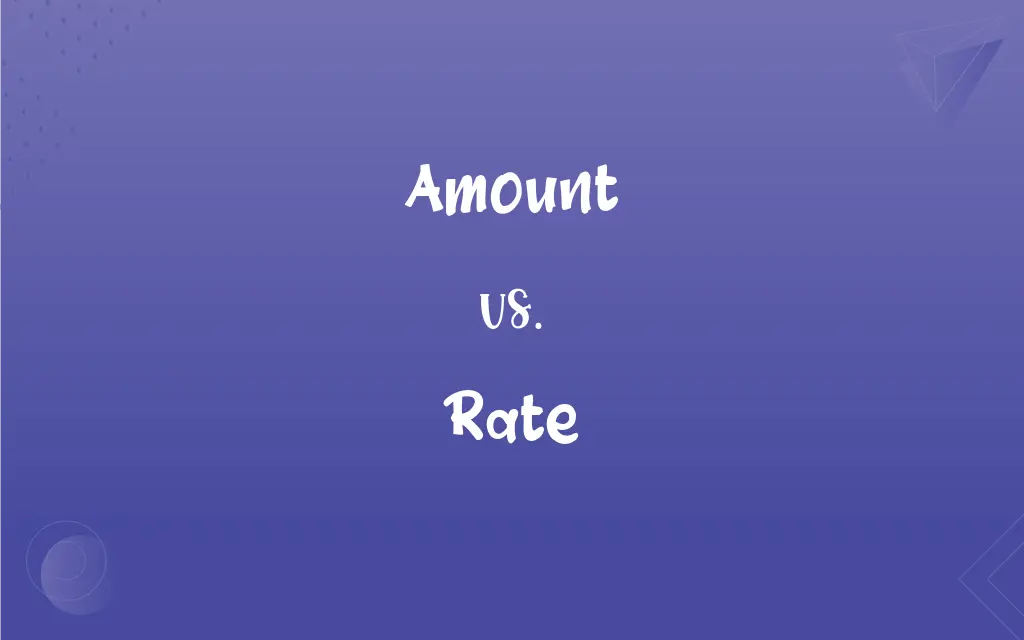# Amount vs. Rate: What's the Difference?Edited by Huma Saeed || By Sumera Saeed || Published on November 16, 2023
Amount means the total quantity or extent of something. Rate means the measure or quantity of something in relation to another quantity or measure.## Key Differences

Amount refers to the total quantity or sum of something, without considering time or rate. Rate, however, measures the frequency or speed of something over a period of time.
When discussing finances, amount could mean the total sum of money, while rate often refers to interest rates or charges per unit time.
Amount is often used to quantify things in bulk or as a whole, such as the amount of water in a tank. Rate usually involves a ratio or comparison, like speed (miles per hour).
In recipes, amount can refer to the quantity of ingredients, whereas rate could be used to describe the rate of pouring or mixing.
Amount can pertain to non-quantifiable aspects, such as the amount of effort. Rate tends to be more quantifiable, like the rate of success.

## Comparison Chart

### Definition

Total quantity
Measure per unit time

### Usage in Finance

Sum of money
Interest or charge per time

### Usage in Measurement

Bulk quantity
Speed or frequency

### Usage in Cooking

Quantity of ingredients
Speed of action (pouring/mixing)

### Quantifiability

Can be non-quantifiable
Usually quantifiable

## Amount and Rate Definitions

#### Amount

Volume or mass.
The amount of water in the tank decreased.

#### Rate

Charge per unit time.
The interest rate was 5% per annum.

#### Amount

Aggregate sum.
The amount of money raised was impressive.

#### Rate

Speed or velocity.
The rate of the car was 60 mph.

#### Amount

Total quantity.
The amount of sugar in the recipe was perfect.

#### Rate

Ratio or proportion.
The rate of exchange fluctuated daily.

#### Amount

Extent or degree.
The amount of effort she put in was commendable.

#### Rate

Measured quantity.
The birth rate increased last year.

#### Amount

Total number.
The amount of people attending surprised them.

#### Rate

Frequency or occurrence.
The rate of growth was steady.

#### Amount

The total of two or more quantities; the aggregate.

#### Rate

A quantity measured with respect to another measured quantity
A rate of speed of 60 miles an hour.

A number; a sum.

#### Rate

A measure of a part with respect to a whole; a proportion
The mortality rate.
A tax rate.

## FAQs

#### Is rate always time-based?

Typically, but it can also be based on other units like distance.

#### Can amount be used for non-tangible things?

Yes, like the amount of love or effort.

#### Is amount used in cooking?

Yes, for ingredient quantities.

#### Can amount be immeasurable?

Sometimes, particularly with abstract concepts.

#### Is the amount used in statistics?

Yes, for totals or sums in data sets.

#### Can rates change over time?

Absolutely, such as fluctuating interest rates.

#### Is amount used in science?

Yes, for quantities in experiments. Rates are used in measuring reactions.

#### Can amount refer to a numerical count?

Yes, it can refer to a total number or count.

#### Is rate important in loans?

Yes, interest rates are crucial in loan agreements.

#### Can amount refer to volume?

Yes, like the amount of liquid in a container.

#### Are rates fixed or variable?

They can be either, depending on the context.

#### Is rate relevant in sports?

Yes, like scoring or running rates.

#### Does amount matter in budgeting?

Definitely, for total expenses or income.

#### Are rates used in demographics?

Yes, like birth or death rates.

#### Do rates apply in physics?

Yes, like rates of acceleration or force.

#### Can amount be vague?

It can be, like saying a large amount.

#### Do rates apply to wages?

Yes, like hourly or annual pay rates.

#### Can amount be subjective?

Sometimes, in describing perceptions.

#### Are rates used in economics?

Yes, for growth rates, inflation rates, etc.